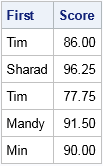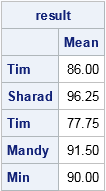Novice SAS programmers quickly learn the advantages of using PROC SORT to sort data, followed by a BY-group analysis of the sorted data. A typical example is to analyze demographic data by state or by ZIP code. A BY statement enables you to produce multiple analyses from a single procedure call.

In the usual BY-group processing of data, the data are sorted by the BY variables. However, there are situations in which you might not want to sort the data. For example, sorting can be an expensive operation for a huge data set. If your data are already grouped by ZIP code, you might want to analyze the data for each ZIP code in the order that they appear in the data set. You can sort the summarized statistics later, if necessary, which will be much faster than sorting the raw data.

SAS supports BY-group analysis of unsorted data. When you construct the BY statement, use the NOTSORTED option to tell a SAS procedure that groups are to be handled in the order in which they appear in the data.

### The NOTSORTED option in the BY statement

The following example uses the NOTSORTED option in the BY statement to analyze data by groups. A teacher has recorded test scores for students in her class. The following DATA step creates 20 observations. There are four observations for each student:

```data Grades; input First \$8. Last \$10. @; do Test = 1 to 4; input Score @; output; end; format ID Z4.; datalines; Tim Albertson 93 89 78 84 Sharad Gupta 100 95 92 98 Tim Williams 85 82 70 74 Mandy Albertson 95 86 90 95 Min Chen 88 92 85 95 ;```

The teacher has committed a serious data-quality mistake: she has failed to include a unique identifier (a "student ID") for her students! Nevertheless, provided that adjacent students in the data set do not share the same first name, the teacher can use the NOTSORTED option in the BY statement to analyze the scores without sorting the data:

```proc means data=Grades noprint; by First notsorted; /* <== use NOTSORTED option by first names */ var Score; output out=Averages mean=; run;   proc print data=Averages noobs; var First Score; run;```The NOTSORTED option tells the procedure to analyze groups that are defined by the FIRST variable. It does not matter that the first names are not sorted or that there are two students named "Tim." The NOTSORTED option prevents the error message:

`ERROR: Data set WORK.GRADES is not sorted in ascending sequence.`

For this example, the data includes the last names of the students, so the teacher could sort the data by last name and first name and use the statement BY Last First to analyze the data. However, the NOTSORTED statement does not require sorted data, which can be a huge advantage. The NOTSORTED option is supported in almost every SAS procedure and DATA step—with the obvious exception of PROC SORT!

For more examples of using the NOTSORTED option in BY-group analyses, see Samudral and Giddings (2006).

### Group processing of unsorted data in SAS/IML

SAS/IML software does not support a BY statement, but you can use various techniques to process groups. The two primary techniques are the UNIQUE-LOC technique and the UNIQUEBY technique. The UNIQUEBY technique can analyze data in the order that they appear, regardless of whether the data are sorted, as shown in the following program:

```proc iml; use Grades; read all var {First Score}; close;   /* the UNIQUEBY function does not require sorted data */ uu = uniqueby(First); /* get first row for each student */ result = j(nrow(uu), 1); /* allocate vector to hold results */ u = uu // (nrow(First)+1); /* trick: append (N+1) to end of indices */ do i = 1 to nrow(u)-1; /* for each group... */ idx = u[i]:(u[i+1]-1); /* get rows in group */ Y = Score[idx]; result[i,] = mean(Y); end;   print result[rowname=(First[uu]) colname="Mean" format=6.2];```In summary, if your data are grouped by an identifying variable, you can analyze the data without sorting. The identifying variable does not have to have unique values. In SAS procedures and the DATA step, you can use the NOTSORTED option in the BY statement. In PROC IML, you can use the UNIQUEBY function.

Share